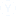Q. How many 3-digit numbers will be there which are divisible by 19?
• PS
• Priya
• 08 jul.
• 262 Visto
• 4 Respuestas4 Respuestas
1-20 of  5000
4 Respuestas
• Let's solve this problem without using any mathematical formula of Arithmetic Progression. This gives us the speedy answer which is so required in the competitive exams where there is a constraint of time.
The greatest three digit number is 999.
And 999/19 = 52.57.
This implies upto 999, a total of 52 numbers are completely divisible.
Subtracting from this, number of two- digit numbers also divisible by 19 taken into account in the previous calculation, i.e 19,38,57,76, and 95, we get :
52-5 = 47
Thus there are 47 such 3 digit numbers in all.

• hmm 47 numbers

• There should be 3 digit number,So First Digit=114
Last digit which is divisible by 19 is 19*52=988

Apply the formula of A.P....An=a+(n-1)d---------------Eq(1)

Where An=988, a=114, common difference d= 19

Applying above value in Eq(1) we get,

988=114+(n-1)19

874=19n-19

893=19n

n=893/19

n=47Otras discusiones relacionadasPractica simulacro de prueba para
Huawei